# Writing a simple custom data viewer¶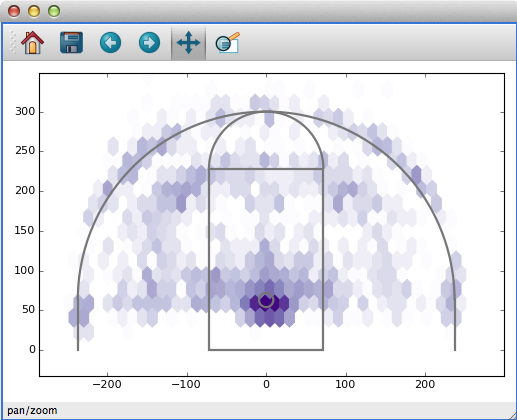Glue’s standard data viewers (scatter plots, images, histograms) are useful in a wide variety of data exploration settings. However, they represent a tiny fraction of the ways to view a particular dataset. For this reason, Glue provides a way to create more data viewers that me better suited to what you need.

There are several ways to do this - the tutorial on this page shows the easiest way for users to develop a new custom visualization, provided that it can be made using Matplotlib and that you don’t want do have to do any GUI programming. If you are interested in building more advanced custom viewers, see Writing a custom viewer for glue with Qt.

## The Goal: Basketball Shot Charts¶

In basketball, Shot Charts show the spatial distribution of shots for a particular player, team, or game. The New York Times has a nice example.

There are three basic features that we might want to incorporate into a shot chart:

• The distribution of shots (or some statistic like the success rate), shown as a heatmap in the background.

• The locations of a particular subset of shots, perhaps plotted as points in the foreground

• The relevant court markings, like the 3-point line and hoop location.

We’ll build a Shot Chart in Glue incrementally, starting with the simplest code that runs.

## Shot Chart Version 1: Heatmap and plot¶

Our first attempt at a shot chart will draw the heatmap of all shots, and overplot shot subsets as points. Here’s the code:

 ``` 1 2 3 4 5 6 7 8 9 10 11 12 13 14 15 16 17 18 19 20 21 22 23 24 25``` ```from glue import custom_viewer from matplotlib.colors import LogNorm bball = custom_viewer('Shot Plot', x='att(x)', y='att(y)') @bball.plot_data def show_hexbin(axes, x, y): axes.hexbin(x, y, cmap='Purples', gridsize=40, norm=LogNorm(), mincnt=1) @bball.plot_subset def show_points(axes, x, y, style): axes.plot(x, y, 'o', alpha=style.alpha, mec=style.color, mfc=style.color, ms=style.markersize) ```

Before looking at the code itself, let’s look at how it’s used. If you include or import this code in your config.py file, Glue will recognize the new viewer. Open this shot catalog, and create a new shot chart with it. You’ll get something that looks like this: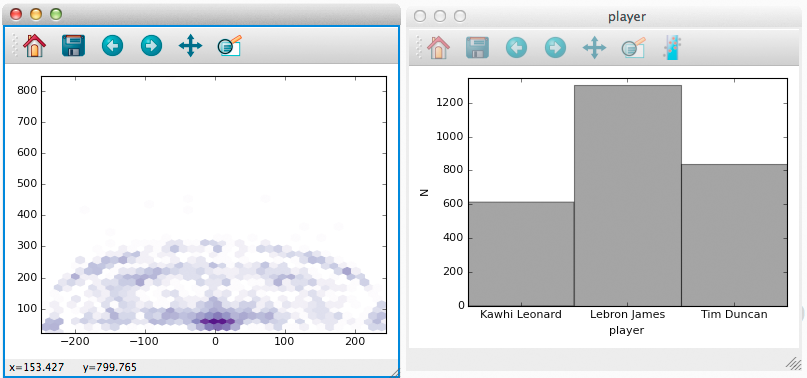Furthermore, subsets that we define (e.g., by selecting regions of a histogram) are shown as points (notice that Tim Duncan’s shots are concentrated closer to the hoop).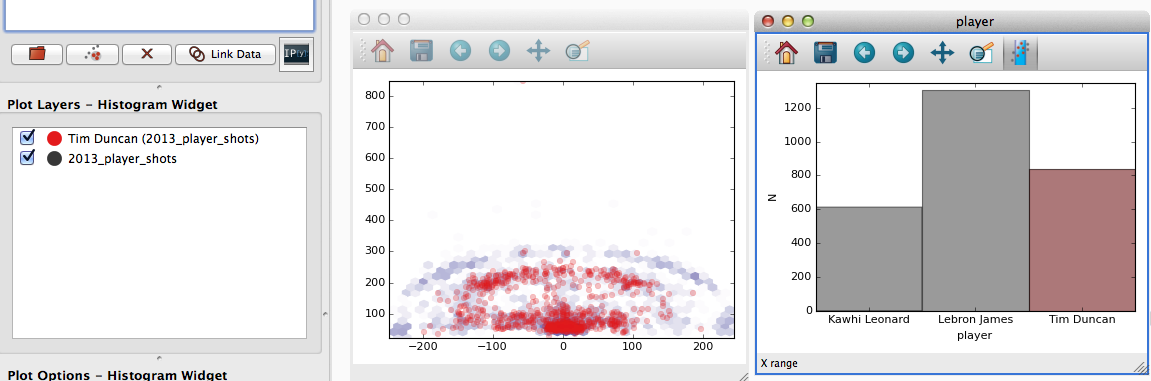Let’s look at what the code does. Line 5 creates a new custom viewer, and gives it the name `Shot Plot`. It also specifies `x` and `y` keywords which we’ll come back to shortly (spoiler: they tell Glue to pass data attributes named `x` and `y` to `show_hexbin`).

Line 11 defines a `show_hexbin` function, that visualizes a dataset as a heatmap. Furthermore, the decorator on line 10 registers this function as the `plot_data` function, responsible for visualizing a dataset as a whole.

Custom functions like `show_hexbin` can accept a variety of input arguments, depending on what they need to do. Glue looks at the names of the inputs to decide what data to pass along. In the case of this function:

• Arguments named `axes` contain the Matplotlib Axes object to draw with

• `x` and `y` were provided as keywords to `custom_viewer`. They contain the data (as arrays) corresponding to the attributes labeled `x` and `y` in the catalog

The function body itself is pretty simple – we just use the `x` and `y` data to build a hexbin plot in Matplotlib.

Lines 19-25 follow a similar structure to handle the visualization of subsets, by defining a `plot_subset` function. We make use of the `style` keyword, to make sure we choose colors, sizes, and opacities that are consistent with the rest of Glue. The value passed to the style keyword is a `VisualAttributes` object.

Custom data viewers give you the control to visualize data how you want, while Glue handles all the tedious bookkeeping associated with updating plots when selections, styles, or datasets change. Try it out!

Still, this viewer is pretty limited. In particular, it’s missing court markings, the ability to select data in the plot, and the ability to interactively change plot settings with widgets. Let’s fix that.

## Shot Chart Version 2: Court markings¶

We’d like to draw court markings to give some context to the heatmap. This is independent of the data, and we only need to render it once. Just as you can register data and subset plot functions, you can also register a setup function that gets called a single time, when the viewer is created. That’s a good place to draw court markings:

 ``` 1 2 3 4 5 6 7 8 9 10 11 12 13 14 15 16 17 18 19 20 21 22 23 24 25 26 27 28 29 30 31 32 33 34 35 36 37 38 39 40 41 42 43 44 45 46 47 48 49 50 51 52``` ```from glue import custom_viewer from matplotlib.colors import LogNorm from matplotlib.patches import Circle, Rectangle, Arc from matplotlib.lines import Line2D bball = custom_viewer('Shot Plot', x='att(x)', y='att(y)') @bball.plot_data def show_hexbin(axes, x, y): axes.hexbin(x, y, cmap='Purples', gridsize=40, norm=LogNorm(), mincnt=1) @bball.plot_subset def show_points(axes, x, y, style): axes.plot(x, y, 'o', alpha=style.alpha, mec=style.color, mfc=style.color, ms=style.markersize) @bball.setup def draw_court(axes): c = '#777777' opts = dict(fc='none', ec=c, lw=2) hoop = Circle((0, 63), radius=9, **opts) axes.add_patch(hoop) box = Rectangle((-6 * 12, 0), 144, 19 * 12, **opts) axes.add_patch(box) inner = Arc((0, 19 * 12), 144, 144, theta1=0, theta2=180, **opts) axes.add_patch(inner) threept = Arc((0, 63), 474, 474, theta1=0, theta2=180, **opts) axes.add_patch(threept) opts = dict(c=c, lw=2) axes.add_line(Line2D([237, 237], [0, 63], **opts)) axes.add_line(Line2D([-237, -237], [0, 63], **opts)) axes.set_ylim(0, 400) axes.set_aspect('equal', adjustable='datalim') ```

This version adds a new `draw_court` function at Line 30. Here’s the result:## Shot Chart Version 3: Widgets¶

There are several parameters we might want to tweak about our visualization as we explore the data. For example, maybe we want to toggle between a heatmap of the shots, and the percentage of successful shots at each location. Or maybe we want to choose the bin size interactively.

The keywords that you pass to `custom_viewer()` allow you to set up this functionality. Keywords serve two purposes: they define new widgets to interact with the viewer, and they define keywords to pass onto drawing functions like `plot_data`.

For example, consider `this version` of the Shot Plot code:

 ``` 1 2 3 4 5 6 7 8 9 10 11 12 13 14 15 16 17 18 19 20 21 22 23 24 25 26 27 28 29 30 31 32 33 34 35 36 37 38 39 40 41 42 43 44 45 46 47 48 49 50 51 52 53 54 55 56 57 58 59 60 61 62 63 64 65``` ```from glue import custom_viewer from matplotlib.colors import LogNorm from matplotlib.patches import Circle, Rectangle, Arc from matplotlib.lines import Line2D import numpy as np bball = custom_viewer('Shot Plot', x='att(x)', y='att(y)', bins=(10, 100), hitrate=False, color=['Reds', 'Purples'], hit='att(shot_made)') @bball.plot_data def show_hexbin(axes, x, y, style, hit, hitrate, color, bins): if hitrate: axes.hexbin(x, y, hit, reduce_C_function=lambda x: np.array(x).mean(), cmap=color, gridsize=bins, mincnt=5) else: axes.hexbin(x, y, cmap=color, gridsize=bins, norm=LogNorm(), mincnt=1) @bball.plot_subset def show_points(axes, x, y, style): axes.plot(x, y, 'o', alpha=style.alpha, mec=style.color, mfc=style.color, ms=style.markersize) @bball.setup def draw_court(axes): c = '#777777' opts = dict(fc='none', ec=c, lw=2) hoop = Circle((0, 63), radius=9, **opts) axes.add_patch(hoop) box = Rectangle((-6 * 12, 0), 144, 19 * 12, **opts) axes.add_patch(box) inner = Arc((0, 19 * 12), 144, 144, theta1=0, theta2=180, **opts) axes.add_patch(inner) threept = Arc((0, 63), 474, 474, theta1=0, theta2=180, **opts) axes.add_patch(threept) opts = dict(c=c, lw=2) axes.add_line(Line2D([237, 237], [0, 63], **opts)) axes.add_line(Line2D([-237, -237], [0, 63], **opts)) axes.set_ylim(0, 400) axes.set_aspect('equal', adjustable='datalim') ```

This code passes 4 new keywords to `custom_viewer()`:

• `bins=(10, 100)` adds a slider widget, to choose an integer between 10 and 100. We’ll use this setting to set the bin size of the heatmap.

• `hitrate=False` adds a checkbox. We’ll use this setting to toggle between a heatmap of total shots, and a map of shot success rate.

• `color=['Reds', 'Purples']` creates a dropdown list of possible colormaps to use for the heatmap.

• `hit='att(shot_made)'` behaves like the x and y keywords from earlier – it doesn’t add a new widget, but it will pass the shot_made data along to our plotting functions.

This results in the following interface: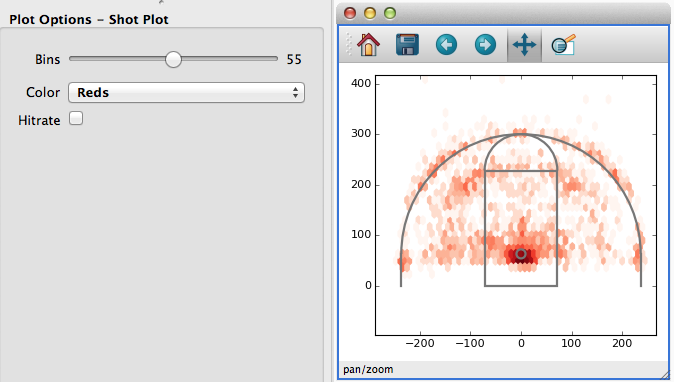Whenever the user changes the settings of these widgets, the drawing functions are re-called. Furthermore, the current setting of each widget is available to the plotting functions:

• `bins` is set to an integer

• `hitrate` is set to a boolean

• `color` is set to `'Reds'` or `'Purples'`

• `x`, `y`, and `hit` are passed as `AttributeWithInfo` objects (which are just numpy arrays with a special `id` attribute, useful when performing selection below).

The plotting functions can use these variables to draw the appropriate plots – in particular, the `show_hexbin` function chooses the binsize, color, and aggregation based on the widget settings.

## Shot Chart Version 4: Selection¶

One key feature still missing from this Shot Chart is the ability to select data by drawing on the plot. To do so, we need to write a `select` function that computes whether a set of data points are contained in a user-drawn `region of interest`:

 ```1 2 3``` ```@bball.select def select(roi, x, y): return roi.contains(x, y) ```

With `this version` of the code you can how draw shapes on the plot to select data: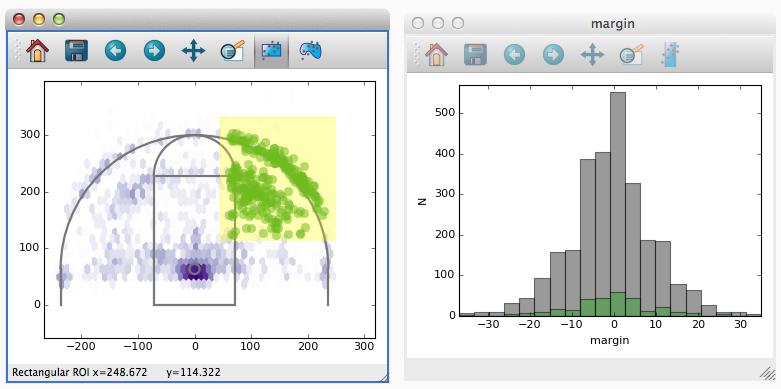## Viewer Subclasses¶

The shot chart example used decorators to define custom plot functions. However, if your used to writing classes you can also subclass `CustomViewer` directly. The code is largely the same:

 ``` 1 2 3 4 5 6 7 8 9 10 11 12 13 14 15 16 17 18 19 20 21 22 23 24 25 26 27 28 29 30 31 32 33 34 35 36 37 38 39 40 41 42 43 44 45 46 47 48 49 50 51 52 53 54 55 56 57 58 59 60 61 62 63 64 65 66 67 68 69 70 71 72``` ```from glue.viewers.custom.qt import CustomViewer from glue.core.subset import RoiSubsetState from matplotlib.colors import LogNorm from matplotlib.patches import Circle, Rectangle, Arc from matplotlib.lines import Line2D import numpy as np class BBall(CustomViewer): name = 'Shot Plot' x = 'att(x)' y = 'att(y)' bins = (10, 100) hitrate = False color = ['Reds', 'Purples'] hit = 'att(shot_made)' def make_selector(self, roi, x, y): state = RoiSubsetState() state.roi = roi state.xatt = x.id state.yatt = y.id return state def plot_data(self, axes, x, y, hit, hitrate, color, bins): if hitrate: axes.hexbin(x, y, hit, reduce_C_function=lambda x: np.array(x).mean(), cmap=color, gridsize=bins, mincnt=5) else: axes.hexbin(x, y, cmap=color, gridsize=bins, norm=LogNorm(), mincnt=1) def plot_subset(self, axes, x, y, style): axes.plot(x, y, 'o', alpha=style.alpha, mec=style.color, mfc=style.color, ms=style.markersize) def setup(self, axes): c = '#777777' opts = dict(fc='none', ec=c, lw=2) hoop = Circle((0, 63), radius=9, **opts) axes.add_patch(hoop) box = Rectangle((-6 * 12, 0), 144, 19 * 12, **opts) axes.add_patch(box) inner = Arc((0, 19 * 12), 144, 144, theta1=0, theta2=180, **opts) axes.add_patch(inner) threept = Arc((0, 63), 474, 474, theta1=0, theta2=180, **opts) axes.add_patch(threept) opts = dict(c=c, lw=2) axes.add_line(Line2D([237, 237], [0, 63], **opts)) axes.add_line(Line2D([-237, -237], [0, 63], **opts)) axes.set_ylim(0, 400) axes.set_aspect('equal', adjustable='datalim') ```

## Valid Function Arguments¶

The following argument names are allowed as inputs to custom viewer functions:

• Any UI setting provided as a keyword to `glue.custom_viewer()`. The value passed to the function will be the current setting of the UI element.

• `axes` is the matplotlib Axes object to draw to

• `roi` is the `glue.core.roi.Roi` object a user created – it’s only available in `make_selection`.

• `style` is available to `plot_data` and `plot_subset`. It is the `VisualAttributes` associated with the subset or dataset to draw

• `state` is a general purpose object that you can use to store data with, in case you need to keep track of state in between function calls.

## UI Elements¶

Simple user interfaces are created by specifying keywords to `custom_viewer()` or class-level variables to `CustomViewer` subclasses. The type of widget, and the value passed to plot functions, depends on the value assigned to each variable. See `custom_viewer()` for information.

## Other Guidelines¶

• You can find other example data viewers at https://github.com/glue-viz/example_data_viewers. Contributions to this repository are welcome!

• Glue auto-assigns the z-order of data and subset layers to the values [0, N_layers - 1]. If you have elements you want to plot in the background, give them a negative z-order

• Glue tries to keep track of the plot layers that each custom function creates, and auto-deletes old layers. This behavior can be disabled by setting `viewer.remove_artists=False`. Likewise, `plot_data` and `plot_subset` can explicitly return a list of newly-created artists. This might be more efficient if your plot is very complicated.

• By default, Glue sets the margins of figures so that the space between axes and the edge of figures is constant in absolute terms. If the default values are not adequate for your viewer, you can set the margins in the `setup` method of the custom viewer by doing e.g.:

```axes.resizer.margins = [0.75, 0.25, 0.5, 0.25]
```

where the list gives the `[left, right, bottom, top]` margins in inches.